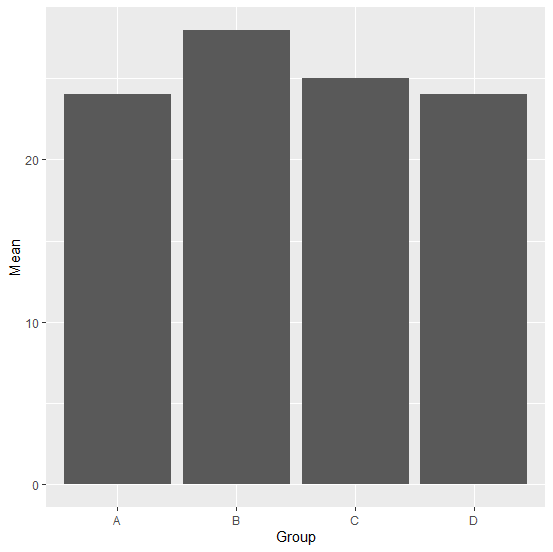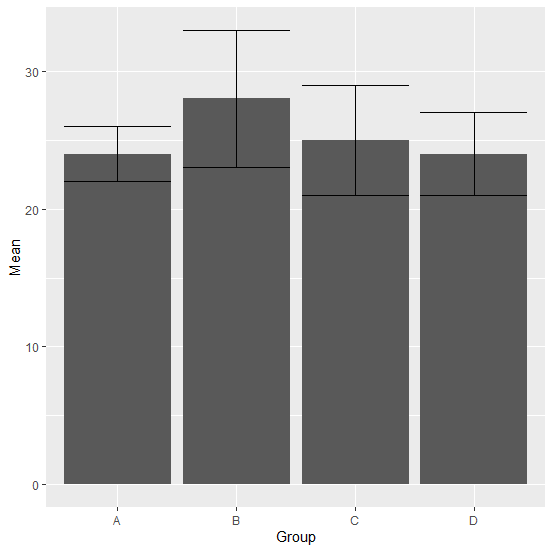# How to create bar plot of means with error bars of standard deviations using ggplot2 in R?

If we have summary data for a group variable then we might want to look at the errors or say differences between mean and standard deviations visually, therefore, we can create a bar plot with error bars of standard deviations. This can be done by using geom_errorbar function of ggplot2 package.

## Example

Consider the below data frame −

Live Demo

Group<-c("A","B","C","D")
Mean<-c(24,28,25,24)
SD<-c(2,5,4,3)
df<-data.frame(Group,Mean,SD)
df

## Output

 Group Mean SD
1  A   24    2
2  B   28    5
3  C   25    4
4  D   24    3

## Example

library(ggplot2)
ggplot(df,aes(Group,Mean))+geom_bar(stat="identity")

## OutputCreating bar plot with error bars of standard deviations −

## Example

ggplot(df,aes(Group,Mean))+geom_bar(stat="identity")+geom_errorbar(aes(x=Group,ym
in=Mean-SD,ymax=Mean+SD))

## OutputUpdated on: 08-Sep-2020

279 Views